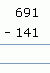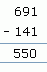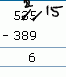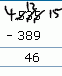Name: ___________________Date:___________________

 Email us to get an instant 20% discount on highly effective K-12 Math & English kwizNET Programs!

### Grade 2 - Mathematics Subtracting - Three Digits

Subtraction without borrow
Method:
1. Start from the units place
2. Subtract the numbers in the units place
3. Subtract the numbers in the tens place
4. Subtract the numbers in the hundreds place
 Question Step 1 Step 2 Step 3Subtraction with borrow
Sometimes when you subtract you need to regroup 1 ten as 10 ones.
 Question Step 1 Step 2 Step 3• Start from the ones place
• In the ones place, since we cannot subtract 9 from 5, we borrow 1 from 3 and make it 2.
• In ones place we get 15-9 = 6
• In the tens place, since we have taken out 1 from 3, we have 3-1=2
• Since we cannot subtract 8 from 2, we borrow 1 from 5 and make it 4.
• In hundreds place, we have 5-3=1Name: ___________________Date:___________________